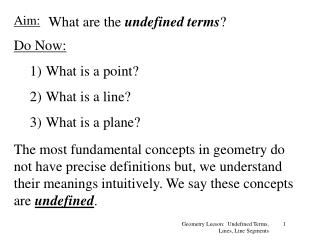DownloadDownload PresentationAim:

# Aim:

Télécharger la présentation## Aim:

- - - - - - - - - - - - - - - - - - - - - - - - - - - E N D - - - - - - - - - - - - - - - - - - - - - - - - - - -
##### Presentation Transcript

1. Aim: What are the undefined terms? • Do Now: • What is a point? • What is a line? • What is a plane? The most fundamental concepts in geometry do not have precise definitions but, we understand their meanings intuitively. We say these concepts are undefined. Geometry Leeson: Undefined Terms, Lines, Line Segments

2. Point: . A(2, 6) Y X A point indicates a position or location in space. . P Points are named using capital letters and/or coordinates. Geometry Leeson: Undefined Terms, Lines, Line Segments

3. Line: Ex: Curved line Ex: Straight line • Naming a Line: • Two points on the line: • Single lowercase letter A B C m A line is an infinite set of adjacent points. Geometry Leeson: Undefined Terms, Lines, Line Segments

4. Plane: • Naming a Plane: • Three points on the plane: • Single uppercase letter: B • C• Plane ABC A • Plane R R A plane is a set of points that forms a completely flat surface. Geometry Leeson: Undefined Terms, Lines, Line Segments

5. Def: Collinear Points: E A B C D A collinear set of points is a set of points all of which lie on the same straight line. • Points A, B, C and D are collinear. • Points A, E and C are notcollinear. Geometry Leeson: Undefined Terms, Lines, Line Segments

6. Def: Line Segment: A B Naming a Line Segment: Use the names of the endpoints. A B “Line segment” is part of “Line” A line segment is the set of two points on a line called endpoints, and all points on the line between the endpoints. Geometry Leeson: Undefined Terms, Lines, Line Segments

7. Measure of Line Segments: To indicate the “measure” of a line segment, use the name of the line segment without the line above the name. Ex: = “measure of line segment” Ex: The perimeter of is 58 cm. If AB = x - 4, BC = 2x + 8, and CA= x – 2, what is BC? The measure of a line segment is the length of the line segment. Ans. BC=36 cm Geometry Leeson: Undefined Terms, Lines, Line Segments

8. Def: Congruent Line Segments: Ex: A B If C D Congruent line segments are segments that are equal in measure. Then AB = CD Ans. CD = 29, AB = 29 Geometry Leeson: Undefined Terms, Lines, Line Segments

9. Def: Midpoint M A B 1) 3) 5) 2) 4) 6) The midpoint of a line segment divides the line segment into two congruent segments. Geometry Leeson: Undefined Terms, Lines, Line Segments

10. Def: Line Bisector Q A B M P 1) 1)  2) The bisector of a line segment is a line that intersects the line segment at its midpoint. X Geometry Leeson: Undefined Terms, Lines, Line Segments

11. Addition and Subtraction of Line Segments: S P R If several line segments belong to the same line, we can write addition and subtraction expressions using the names of these segments. Geometry Leeson: Undefined Terms, Lines, Line Segments

12. Simple 2-Column Proof B P F E A Given Definition of Line Bisector Definition of Midpoint Geometry Leeson: Undefined Terms, Lines, Line Segments